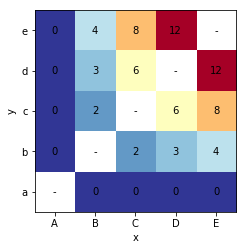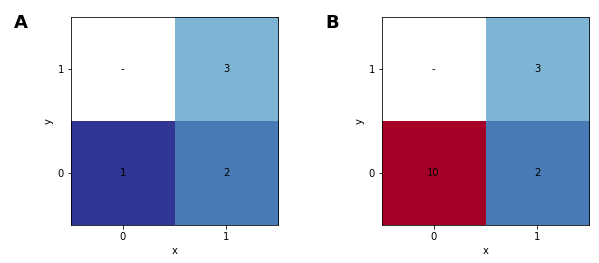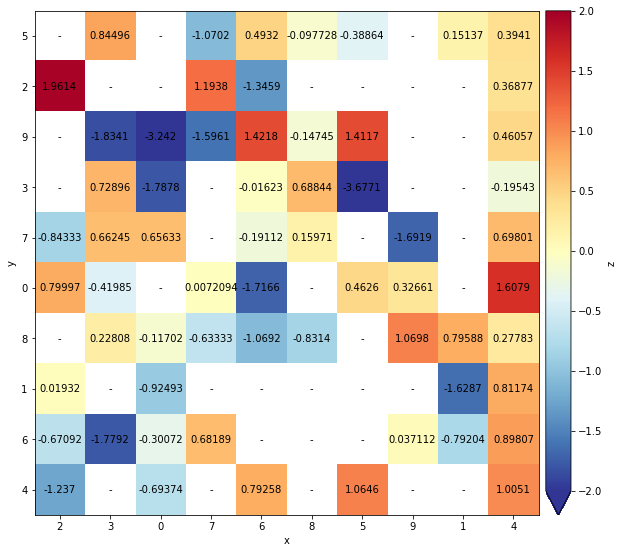# Heatmap¶

Title
HeatMap Element
Dependencies
Matplotlib
Backends
Matplotlib
Bokeh
In :
import numpy as np
import holoviews as hv
hv.extension('matplotlib')HeatMap visualises tabular data indexed by two key dimensions as a grid of colored values. This allows spotting correlations in multivariate data and provides a high-level overview of how the two variables are plotted.

The data for a HeatMap may be supplied as 2D tabular data with one or more associated value dimensions. The first value dimension will be colormapped, but further value dimensions may be revealed using the hover tool.

In :
data = [(chr(65+i), chr(97+j),  i*j) for i in range(5) for j in range(5) if i!=j]
hv.HeatMap(data).sort()

Out:It is important to note that the data should be aggregated before plotting as the HeatMap cannot display multiple values for one coordinate and will simply use the first value it finds for each combination of x- and y-coordinates.

In :
heatmap = hv.HeatMap([(0, 0, 1), (0, 0, 10), (1, 0, 2), (1, 1, 3)])
heatmap + heatmap.aggregate(function=np.max)

Out:As the above example shows before aggregating the second value for the (0, 0) is ignored unless we aggregate the data first.

To reveal the values of a HeatMap we can enable a colorbar and if you wish to have interactive hover information, you can use the hover tool in the Bokeh backend:

In :
heatmap = hv.HeatMap((np.random.randint(0, 10, 100), np.random.randint(0, 10, 100),
np.random.randn(100), np.random.randn(100)), vdims=['z', 'z2']).redim.range(z=(-2, 2))

heatmap.opts(colorbar=True, fig_size=250)

Out:For full documentation and the available style and plot options, use hv.help(hv.HeatMap).